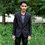# Limit of a division of products

$\large\lim_{n \to\infty} \dfrac{\displaystyle\prod_{r=0}^n{(2r+1)}}{\displaystyle\prod_{r=1}^{n+1}{2r}}$

I am unable to compute this limit mathematically, but my guess is that it is $0$. Please post your solution to this.Note by Swagat Panda
4 years, 11 months ago

This discussion board is a place to discuss our Daily Challenges and the math and science related to those challenges. Explanations are more than just a solution — they should explain the steps and thinking strategies that you used to obtain the solution. Comments should further the discussion of math and science.

When posting on Brilliant:

• Use the emojis to react to an explanation, whether you're congratulating a job well done , or just really confused .
• Ask specific questions about the challenge or the steps in somebody's explanation. Well-posed questions can add a lot to the discussion, but posting "I don't understand!" doesn't help anyone.
• Try to contribute something new to the discussion, whether it is an extension, generalization or other idea related to the challenge.

MarkdownAppears as
*italics* or _italics_ italics
**bold** or __bold__ bold
- bulleted- list
• bulleted
• list
1. numbered2. list
1. numbered
2. list
Note: you must add a full line of space before and after lists for them to show up correctly
paragraph 1paragraph 2

paragraph 1

paragraph 2

[example link](https://brilliant.org)example link
> This is a quote
This is a quote
    # I indented these lines
# 4 spaces, and now they show
# up as a code block.

print "hello world"
# I indented these lines
# 4 spaces, and now they show
# up as a code block.

print "hello world"
MathAppears as
Remember to wrap math in $$ ... $$ or $ ... $ to ensure proper formatting.
2 \times 3 $2 \times 3$
2^{34} $2^{34}$
a_{i-1} $a_{i-1}$
\frac{2}{3} $\frac{2}{3}$
\sqrt{2} $\sqrt{2}$
\sum_{i=1}^3 $\sum_{i=1}^3$
\sin \theta $\sin \theta$
\boxed{123} $\boxed{123}$

Sort by:

use sandwich theorem , it greatly helps here

- 4 years, 11 months ago

Thanks very much for the suggestion, but I don't know how to apply sandwich theorem in problems, I have only studied it in theory, can you give me a hint? :)

- 4 years, 11 months ago

The infinite product representation of $\sin x$ is $\displaystyle \frac{\sin x}{x} = \prod_{r=1}^{\infty} (1-\frac{x^2}{r^2 \pi^2} )$

Put $\displaystyle x=\frac{\pi}{2}$ to check that , $\displaystyle \frac{2}{\pi}=\frac{1.3.3.5.5.7.7\cdots}{2.2.4.4.6.6\cdots} = (\frac{1.3.5\cdots}{2.4.6\cdots})^2$

So your limit is : $\displaystyle \prod_{n=1}^{\infty} \frac{2n-1}{2n} = \sqrt{\frac{2}{\pi}}$

- 4 years, 11 months ago

Okay, but my question is different from your expression in the last step, you have solved for $\displaystyle\prod_{n=1}^{\infty}{\frac{2n-1}{2n}}$ ,whereas I asked for $\displaystyle\lim_{n \rightarrow \infty} \frac{\displaystyle\prod_{r=0}^n{(2r+1)}}{\displaystyle\prod_{r=1}^{n+1}{2r}}$

- 4 years, 11 months ago

Observe that $\prod_{r=0}^{n} (2r+1)=\prod_{r=1}^{n}(2(r-1)+1)=\prod_{r=1}^{n}(2r-1)$ , Put limit to obtain $\displaystyle \lim_{n\to\infty}\prod_{r=0}^{n}(2r+1)=\prod_{r=1}^{\infty}(2n-1)$

Similarly , $\displaystyle \lim_{n\to\infty}\prod_{r=1}^{n+1}2r=\prod_{r=1}^{\infty}2r$

- 4 years, 11 months ago

I think the answer is $0$ because, $\displaystyle\lim _{ n\rightarrow \infty } \dfrac \prod _{ r=0 }^{ n }{ (2r+1) } }{ \displaystyle\prod _{ r=1 }^{ n+1 }{ 2r } } =\displaystyle\lim _{ n\rightarrow \infty } \dfrac { 1\cdot 3\cdot 5\cdots (2n+1) }{ 2\cdot 4\cdot 6\cdots (2(n+1)) }$ Multiplying both sides by $\pi/2$, and using Walli's formula, we get: $\displaystyle\lim _{ n\rightarrow \infty } \dfrac\pi2\cdot\dfrac { 1\cdot 3\cdot 5\cdots (2n+1) }{ 2\cdot 4\cdot 6\cdots (2(n+1)) }=\displaystyle\lim _{ n\rightarrow \infty } \displaystyle \int_{0}^{{\pi}/{2}} {\sin ^{ 2n+2 }{ x }.dx }=\displaystyle\lim _{ n\rightarrow \infty }\dfrac{\Gamma \left({\dfrac32+n}\right)}{\sqrt π\cdot \Gamma{\left(2+n\right) }} =\boxed0 \\ \Rightarrow \displaystyle\lim _{ n\rightarrow \infty } \dfrac \prod _{ r=0 }^{ n }{ (2r+1) } }{ \displaystyle\prod _{ r=1 }^{ n+1 }{ 2r } }=\dfrac2\pi \times\left(\displaystyle\lim _{ n\rightarrow \infty }\dfrac{\sqrt π \cdot \Gamma \left({\dfrac32+n}\right)}{2\cdot \Gamma{\left(2+n\right) }}\right)=\dfrac2\pi \times 0 =\boxed0$

I used wolfram alpha for computing the second last step, because I don't know how to compute limits in gamma functions.

The given function can also be written as

$\displaystyle\lim_{n \rightarrow \infty}\dfrac{(2n+2)!}{\left(2^{n+1}\cdot(n+1)!\right)^{2}}=\displaystyle\lim_{n \rightarrow \infty}\dfrac {\dbinom{2n+2}{n+1} } { (2^{ n+1 })^{ 2 } }=0$ because$\dfrac {\dbinom{2n+2}{n+1} }{ (2^{ n+1 })^{ 2 } }$ is a decreasing function.

- 4 years, 11 months ago

Yes, this is correct. The limit is 0. However, instead of using W|A for calculating the limit, you could use Legendre's duplication formula to get $2^{2n+2}\Gamma(n+\frac 32)\Gamma(n+2)=\sqrt{\pi}\cdot\Gamma(2n+3)$ and using this along with $\dfrac{\Gamma(2n+3)}{\left(\Gamma(n+2)\right)^2}=\dfrac{(2n+2)!}{\left((n+1)!\right)^2}=\dbinom{2n+2}{n+1}$, you could rewrite the limit as,

$L=\lim_{n\to\infty}\frac{\Gamma(n+3/2)}{\sqrt\pi\cdot\Gamma(n+2)}=\lim_{n\to\infty}\frac{\Gamma(2n+3)}{2^{2n+2}\cdot\left(\Gamma(n+2)\right)^2}=\lim_{n\to\infty}\frac{\binom{2n+2}{n+1}}{(2^{n+1})^2}$

Note that this is the second limit you mentioned in your comment. Rather than concluding that the limit is 0 using a handwavy argument that the binomial coefficient is a decreasing function (I can't see an easy way to verify it), I think a formal way would be to use a corollary of Stirling's result that $\dbinom{2n}n\sim\dfrac{4^n}{\sqrt{\pi n}}$ as $n\to\infty$, so the limit becomes,

$L=\lim_{n\to\infty}\frac{4^{n+1}}{\sqrt{\pi(n+1)}(2^{n+1})^2}=\frac 1{\sqrt\pi}\lim_{n\to\infty}\frac{1}{\sqrt{n+1}}=0$

where the penultimate limit is easily evaluated noting that $\sqrt{n+1}\to\infty$ as $n\to\infty$, hence $\frac{1}{\sqrt{n+1}}\to 0$ as $n\to\infty$.

- 4 years, 9 months ago

I asked for help because I couldn't find a way to interpret this limit formally using methods mentioned in the JEE syllabus and I was relatively new to Stirling's approximation then, which is why I gave the reason of decreasing function. Thanks for helping me out.

Also you don't need to go to Legendre's formula to get to the function I mentioned. Just multiply the numerator and denominator with the denominator(i.e. product of even numbers) again to get that.

- 4 years, 9 months ago

This is incorrect. Putting $x=\pi/2$ gives $\dfrac 2\pi=\prod\limits_{n=1}^\infty\dfrac{(2n)^2-1}{(2n)^2}$. So, we have $\sqrt{\dfrac 2\pi}=\prod\limits_{n=1}^\infty\dfrac{\sqrt{(2n)^2-1}}{2n}$ which is obviously not the same as the one you wrote.

I guess you mistook the numerator $(2n)^2-1$ as $(2n-1)^2$.

- 4 years, 9 months ago